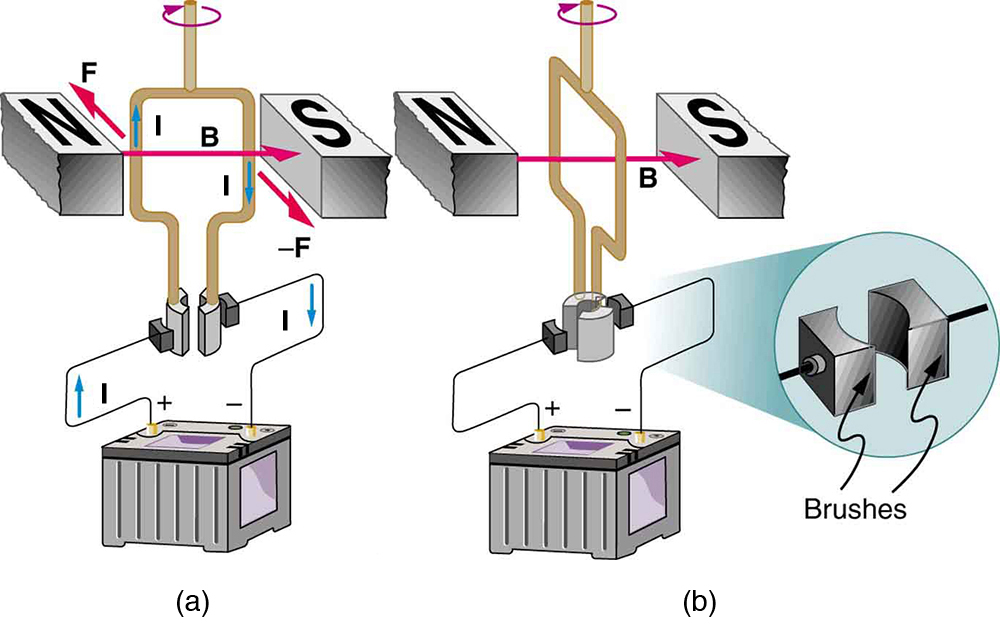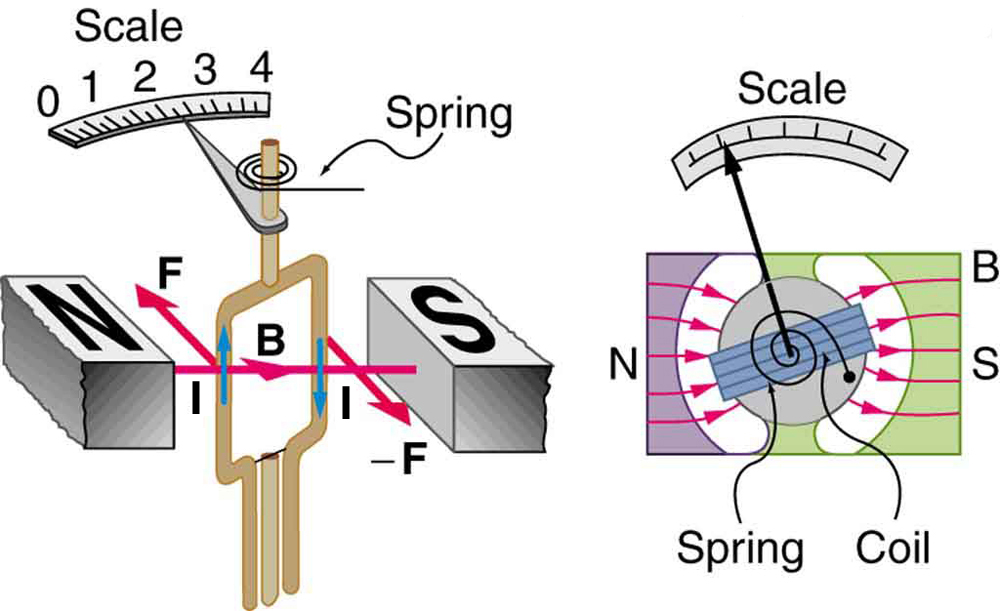# 22.8 Torque on a current loop: motors and meters  (Page 2/4)

 Page 2 / 4

The torque found in the preceding example is the maximum. As the coil rotates, the torque decreases to zero at $\theta =0$ . The torque then reverses its direction once the coil rotates past $\theta =0$ . (See [link] (d).) This means that, unless we do something, the coil will oscillate back and forth about equilibrium at $\theta =0$ . To get the coil to continue rotating in the same direction, we can reverse the current as it passes through $\theta =0$ with automatic switches called brushes . (See [link] .)(a) As the angular momentum of the coil carries it through θ = 0 size 12{θ=0} {} , the brushes reverse the current to keep the torque clockwise. (b) The coil will rotate continuously in the clockwise direction, with the current reversing each half revolution to maintain the clockwise torque.

Meters , such as those in analog fuel gauges on a car, are another common application of magnetic torque on a current-carrying loop. [link] shows that a meter is very similar in construction to a motor. The meter in the figure has its magnets shaped to limit the effect of $\theta$ by making $B$ perpendicular to the loop over a large angular range. Thus the torque is proportional to $I$ and not $\theta$ . A linear spring exerts a counter-torque that balances the current-produced torque. This makes the needle deflection proportional to $I$ . If an exact proportionality cannot be achieved, the gauge reading can be calibrated. To produce a galvanometer for use in analog voltmeters and ammeters that have a low resistance and respond to small currents, we use a large loop area $A$ , high magnetic field $B$ , and low-resistance coils.Meters are very similar to motors but only rotate through a part of a revolution. The magnetic poles of this meter are shaped to keep the component of B size 12{B} {} perpendicular to the loop constant, so that the torque does not depend on θ size 12{θ} {} and the deflection against the return spring is proportional only to the current I size 12{I} {} .

## Section summary

• The torque $\tau$ on a current-carrying loop of any shape in a uniform magnetic field. is
$\tau =\text{NIAB}\phantom{\rule{0.25em}{0ex}}\text{sin}\phantom{\rule{0.25em}{0ex}}\theta ,$
where $N$ is the number of turns, $I$ is the current, $A$ is the area of the loop, $B$ is the magnetic field strength, and $\theta$ is the angle between the perpendicular to the loop and the magnetic field.

## Conceptual questions

Draw a diagram and use RHR-1 to show that the forces on the top and bottom segments of the motor’s current loop in [link] are vertical and produce no torque about the axis of rotation.

## Problems&Exercises

(a) By how many percent is the torque of a motor decreased if its permanent magnets lose 5.0% of their strength? (b) How many percent would the current need to be increased to return the torque to original values?

(a) $\text{τ}$ decreases by 5.00% if B decreases by 5.00%

(b) 5.26% increase

(a) What is the maximum torque on a 150-turn square loop of wire 18.0 cm on a side that carries a 50.0-A current in a 1.60-T field? (b) What is the torque when $\theta$ is $\text{10}\text{.}9º?$

Find the current through a loop needed to create a maximum torque of $9\text{.}\text{00 N}\cdot \text{m.}$ The loop has 50 square turns that are 15.0 cm on a side and is in a uniform 0.800-T magnetic field.

10.0 A

Calculate the magnetic field strength needed on a 200-turn square loop 20.0 cm on a side to create a maximum torque of $\text{300 N}\cdot \text{m}$ if the loop is carrying 25.0 A.

Since the equation for torque on a current-carrying loop is $\tau =\text{NIAB}\phantom{\rule{0.25em}{0ex}}\text{sin}\phantom{\rule{0.25em}{0ex}}\theta$ , the units of $N\cdot m$ must equal units of $A\cdot {m}^{2}\phantom{\rule{0.25em}{0ex}}T$ . Verify this.

$A\cdot {m}^{2}\cdot T=A\cdot {m}^{2}\left(\frac{N}{A\cdot m}\right)=N\cdot m$ .

(a) At what angle $\theta$ is the torque on a current loop 90.0% of maximum? (b) 50.0% of maximum? (c) 10.0% of maximum?

A proton has a magnetic field due to its spin on its axis. The field is similar to that created by a circular current loop $0\text{.}\text{650}×{\text{10}}^{-\text{15}}\phantom{\rule{0.25em}{0ex}}m$ in radius with a current of $1\text{.}\text{05}×{\text{10}}^{4}\phantom{\rule{0.25em}{0ex}}A$ (no kidding). Find the maximum torque on a proton in a 2.50-T field. (This is a significant torque on a small particle.)

$3\text{.}\text{48}×{\text{10}}^{-\text{26}}\phantom{\rule{0.25em}{0ex}}N\cdot m$

(a) A 200-turn circular loop of radius 50.0 cm is vertical, with its axis on an east-west line. A current of 100 A circulates clockwise in the loop when viewed from the east. The Earth’s field here is due north, parallel to the ground, with a strength of $3\text{.}\text{00}×{\text{10}}^{-5}\phantom{\rule{0.25em}{0ex}}T$ . What are the direction and magnitude of the torque on the loop? (b) Does this device have any practical applications as a motor?

Repeat [link] , but with the loop lying flat on the ground with its current circulating counterclockwise (when viewed from above) in a location where the Earth’s field is north, but at an angle $\text{45}\text{.}0º$ below the horizontal and with a strength of $\text{6.}\text{00}×{\text{10}}^{-5}\phantom{\rule{0.25em}{0ex}}T$ .

(a) $\text{0.666 N}\cdot m$ west

(b) This is not a very significant torque, so practical use would be limited. Also, the current would need to be alternated to make the loop rotate (otherwise it would oscillate).

#### Questions & Answers

a15kg powerexerted by the foresafter 3second
Firdos Reply
what is displacement
Xolani Reply
movement in a direction
Jason
Explain why magnetic damping might not be effective on an object made of several thin conducting layers separated by insulation? can someone please explain this i need it for my final exam
anas Reply
Hi
saeid
hi
Yimam
What is thê principle behind movement of thê taps control
Oluwakayode Reply
what is atomic mass
thomas Reply
this is the mass of an atom of an element in ratio with the mass of carbon-atom
Chukwuka
show me how to get the accuracies of the values of the resistors for the two circuits i.e for series and parallel sides
Jesuovie Reply
Explain why it is difficult to have an ideal machine in real life situations.
Isaac Reply
tell me
Promise
what's the s . i unit for couple?
Promise
its s.i unit is Nm
Covenant
Force×perpendicular distance N×m=Nm
Oluwakayode
İt iş diffucult to have idêal machine because of FRİCTİON definitely reduce thê efficiency
Oluwakayode
if the classica theory of specific heat is valid,what would be the thermal energy of one kmol of copper at the debye temperature (for copper is 340k)
Zaharadeen Reply
can i get all formulas of physics
BPH Reply
yes
haider
what affects fluid
Doreen Reply
pressure
Oluwakayode
Dimension for force MLT-2
Promise Reply
what is the dimensions of Force?
Osueke Reply
how do you calculate the 5% uncertainty of 4cm?
melia Reply
4cm/100×5= 0.2cm
haider
how do you calculate the 5% absolute uncertainty of a 200g mass?
melia Reply
= 200g±(5%)10g
haider
use the 10g as the uncertainty?
melia
which topic u discussing about?
haider
topic of question?
haider
the relationship between the applied force and the deflection
melia
sorry wrong question i meant the 5% uncertainty of 4cm?
melia
its 0.2 cm or 2mm
haider
thank you
melia
Hello group...
Chioma
hi
haider
well hello there
sean
hi
Noks
hii
Chibueze
10g
Olokuntoye
0.2m
Olokuntoye
hi guys
thomas
the meaning of phrase in physics
Chovwe Reply
is the meaning of phrase in physics
Chovwe

### Read also:

#### Get Jobilize Job Search Mobile App in your pocket Now!

Source:  OpenStax, College physics. OpenStax CNX. Jul 27, 2015 Download for free at http://legacy.cnx.org/content/col11406/1.9
Google Play and the Google Play logo are trademarks of Google Inc.

Notification Switch

Would you like to follow the 'College physics' conversation and receive update notifications?ByByBy RhodesBy Anonymous UserBy OpenStaxBy OpenStaxBy Edgar DelgadoBy OpenStaxBy Brooke DelaneyBy OpenStaxBy OpenStaxBy Sarah Warren